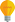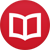# How to quickly form any interval from any note

by Jakub MarianTip: See my list of theMost Common Mistakes in English. It will teach you how to avoid mis­takes with com­mas, pre­pos­i­tions, ir­reg­u­lar verbs, and much more.

Using the traditional approach, if you want to be able to quickly name any interval from any note, you basically have to memorize them all; needless to say, this is a huge amount of data.

If you have some time for thinking, you can memorize the intervals between the notes A, B, …, G (without accidentals) and then derive the others by memorizing how accidentals change the intervals (for example, if you put a sharp in front of the bottom note of an interval, it ‘shrinks’, i.e. the major third becomes the minor third, the minor third becomes the diminished third etc.).

Both of these approaches are highly ineffective (in terms of the time/naming ability ratio). In this article, I am going to teach you my own approach using which you will be able to name any interval quickly (and also analyse any interval quickly) by memorizing only a tiny fraction of the amount of data you would have to memorize using the traditional approach.

This approach is part of a larger theory, called The Numeral Notation, which is essentially a complete approach to harmony theory (especially to formation and analysis of chord progressions) that can be learned without much memorization (unlike the traditional theory).

The first step is to memorize the following table (the interval between two neighbouring notes is always a perfect fifth):

 F C G D A E B -3 -2 -1 0 1 2 3

To get the numerical representation of notes with sharps and flats, simply remember the rule that:

♯ (sharp) means “+7”, b (flat) means “–7”. For example, F = –3, so F♯ = –3+7 = 4, and A = 1, so Ab = 1–7 = –6.

Now it is easy to remember the whole table:

 Fb Cb Gb Db Ab Eb Bb F C G D A E B F♯ C♯ G♯ D♯ A♯ E♯ B♯ -10 -9 -8 -7 -6 -5 -4 -3 -2 -1 0 1 2 3 4 5 6 7 8 9 10

Double flats and sharps are relatively rare, and it may not be worth the effort to memorize the corresponding numbers, but you can always calculate the numbers easily using the rule above. For example, A = 1, A♯ = 1 + 7 = 8, Ax = “A♯♯” = 8 + 7 = 15.

## Intervals

Every interval corresponds to a certain difference in the numerical representation. Let’s first show a few examples to make the concept clear. The major third above a note is “+4”, so the major thirds above the following notes are:

Db + 4 = –7 + 4 = –3 = F
C + 4 = –2 + 4 = 2 = E
A + 4 = 1 + 4 = 5 = C♯
F♯ + 4 = 4 + 4 = 8 = A♯

If you memorize the table above, making such calculations in your head will be completely trivial because you are just adding small numbers. The best way to do that is, in my opinion, to think about the notes as different names for the numbers. If you were learning French, you would learn that 1, 2, 3 are called un, deux, troisand similarly, they are called A, E, B in the language of music.

Intervals in the numeral notation need not always be positive. For example, the minor third above a note is “–3” (one way to see that is that the minor third is the major third with a flat sign, i.e. +4–7 = –3). Calculating the minor thirds from the same notes as in the example above would give:

Db – 3 = –7 – 3 = –10 = Fb
C – 3 = –2 – 3 = –5 = Eb
A – 3 = 1 – 3 = –2 = C
F♯ – 3 = 4 – 3 = 1 = A

The sign is important. “X – 3” is a minor third above X, whereas “X + 3” would be a minor third below X.

Now, if you want to be able to form all intervals using their traditional names, you will have to memorize the following table:

 Interval Alternative name Value Minor second up Major seventh down –5 Major second up Minor seventh down +2 Minor third up Major sixth down –3 Major third up Minor sixth down +4 Perfect fourth up Perfect fifth down –1 Perfect fifth up Perfect fourth down +1 Minor sixth up Major third down –4 Major sixth up Minor third down +3 Minor seventh up Major second down –2 Major seventh up Minor second down +5

However, in practice as a musician, you don’t usually think about the names of intervals; you think about larger harmonic concepts. One such concept could be the major triad, which consists of a root, the major third above it, and the perfect fifth above it. In the numeral notation, this would be X, X+4, and X+1. For example, let’s form the major triads on Db = –7, C = –2, A = 1, and F♯ = 4:

–7 –3 –6 = Db F Ab
–2 2 –1 = C E G
1 5 2 = A C♯ E
4 8 5 = F♯ A♯ C♯

Once you start thinking about the numbers using the names given above, the rest comes automatically. When someone asks you what notes are in the E major triad, what you really hear in your mind is the number 2, so you immediately see that the triad is “2 6 3” (that’s just trivial arithmetic), and you say the names of these numbers in the musical language, i.e. E G♯ B.

By the way, I have written several educational ebooks. If you get a copy, you can learn new things and support this website at the same time—why don’t you check them out?